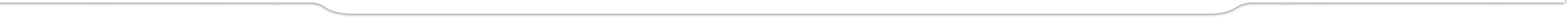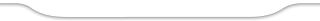# Tools

## Tracking System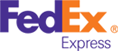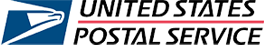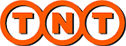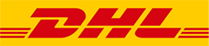## Dimensional Weight Calculator

Enter Dimension
Your weight: 0.00 lb or 0.00 kg
Select Unit
Dimensional weight is calculated by length *
height * width of a package , in inches,
and dividing by 139.

Dimensional Weight = W * H * L / 139Example 1: Laptop Box
Dimension:
45 * 30 * 11 (cm)
Dimension Weight:
7 (lb)
Actual Weight:
10 (lb)
Billable Weight:
10 (lb)Example 2: Shoe Box
Dimension:
14.3 * 7.5 * 5.15 (inch)
Dimension Weight:
6 (lb)
Actual Weight:
5 (lb)
Billable Weight:
6 (lb)Example 3: Pillow
Dimension:
14.3 * 7.5 * 5.15 (inch)
Dimension Weight:
6 (lb)
Actual Weight:
5 (lb)
Billable Weight:
6 (lb)

Equals to